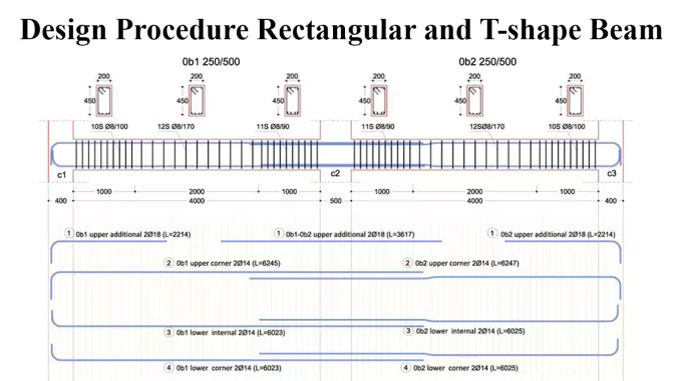# How to design rectangular and T shape beamBeams are defined as members which are exposed to flexure. So, it is important to give attention to the analysis of bending moment, shear and deflection.

When the bending moment operates on the beam, bending strain is created. The resisting moment is formed with internal stresses. Under positive moment, compressive strains are developed in the top of the beam and tensile strains in the bottom.

Concrete is weak against tensile strength and it is not perfect for flexure member by itself. The tension side of the beam will collapse prior to failure of compression side when beam is exposed to a bending moment devoid of the reinforcement. To resolve this issue, steel reinforcement is provided on the tension side. The steel reinforcement withstands all tensile bending stress as tensile strength of concrete is zero when cracks are formed.

Rectangular beam

Accept the depth of beam with the ACI code reference, least thickness until the deflection is considered.
Accept the beam width (ratio of width and depth is approx 1:2).

Calculate self-weight of beam & design load.
Work out factored load (1.4 DL + 1.7 LL).
Calculate design moment (Mu)
Work out maximum possible nominal moment for singly reinforced beam (fM n ).

Determine reinforcement type by making comparison between the design moment (M u ) and the maximum possible moment for the singly reinforced beam (fM n ). If fM n remains under Mu, the beam should be designed as a doubly reinforced beam otherwise the beam should be designed with tension steel only.

Find out the moment strength of the singly reinforced section (concrete-steel couple).

Calculate the necessary steel area for the singly reinforced section.
Determine an essential residual moment, deducting the total design moment and the moment capacity of the singly reinforced section.
Calculate the extra steel area from the required residual moment.
Calculate the total tension and compressive steel area.
Design the reinforcement with the selection of the steel.
Verify the actual beam depth and assumed beam depth.

T-shape Beam

Calculate the design moment (Mu ).
Presume the effective depth.
Choose the effective flange width (b) depending on ACI criteria.

Workout the practical moment strength (fM n ) anticipating the total effective flange is supporting the compression.

When the practical moment strength (fM n ) is greater than the design moment (Mu ), the beam is measured as a rectangular T-beam with the effective flange width b. If the practical moment strength (fM n ) is not more than the design moment (Mu ), the beam will operate as a true T-shape beam.

Determine the approximate lever arm distance for the internal couple.
Work out the approximate required steel area.

Design the reinforcement
Verify the beam width
Calculate the actual effective depth and analyze the beam